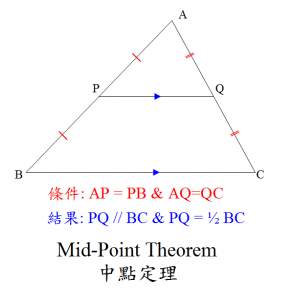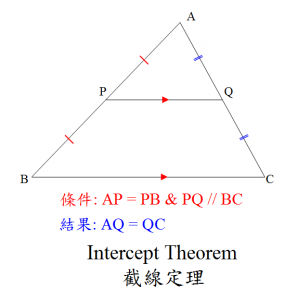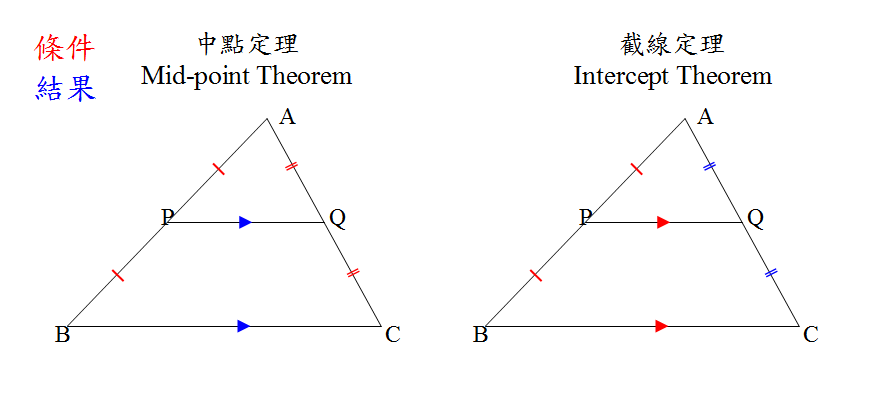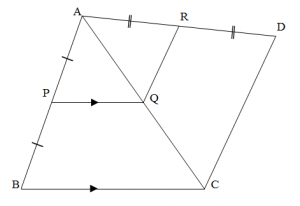# 中點定理和截線定理

• 27/06/2011Prove AQR = ∠ACD.Solution:

##\text{In }\triangle ABC,\\
\\
\because AP=PB\ \text{and}\ PQ// BC\\
\therefore AQ = QC\ \text{(Intercept Theorem)}##

##\text{In }\triangle ACD,\\
\\
\because AQ=QC\ \text{and}\ AR = RD\\
\therefore QR\ //\ CD\ \text{(Mid-Point Theorem)}##

##\therefore \angle AQR = \angle ACD\ (corr. \angle s,\ QR // CD)##

### 回應 (6)

1.Fonz Tsui 說：

Thanks Thomas, very good materials for teaching my daughter maths

2.Yip Shun 說：

根據截線定理 的 圖, AP = PB 及PQ//BC, 如果要證明BC = 2PQ, 該結果不能被稱為 截線定理? 要先用證AQ = QC再用一次中點定理? 此方法, 或者證明 ΔABC 和 ΔAPQ 相似。然後再引用對應邊成比例, 都似乎太慢.

3.ndjfc 說：

hi

4.K.jr 說：

converse of mid-point theorem……why wont the teachers teach that as well……darn…

5.Mr.Jason Sason 說：

basic

6.Derek Poon 說：

句子“運用中點定理的先決條件是 AP = PM”有誤，應該是 AP = PB